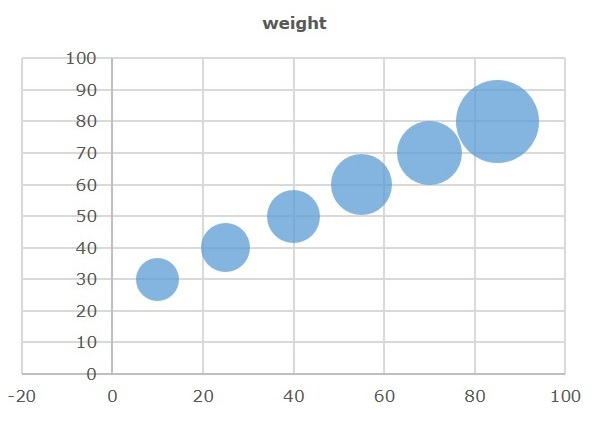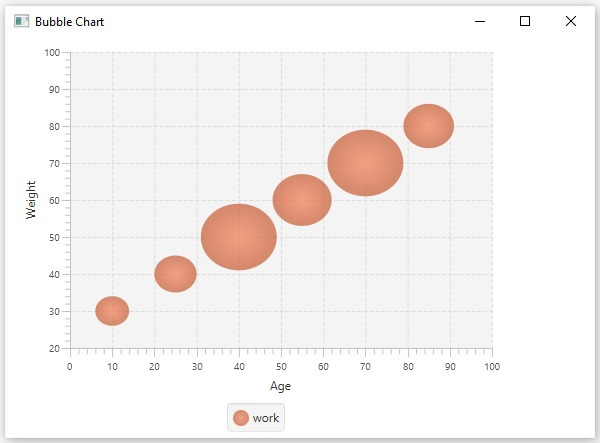# JavaFX - Bubble Chart

A bubble chart is used to plant three-dimensional data; the third dimension will be represented by the size (radius) of the bubble.

The following is a Bubble chart depicting the work done.In JavaFX, a Bubble chart is represented by a class named BubbleChart. This class belongs to the package javafx.scene.chart. By instantiating this class, you can create an BubbleChart node in JavaFX.

## Steps to Generate Bubble Chart

To generate a bubble chart in JavaFX, follow the steps given below.

### Step 1: Creating a Class

Create a Java class and inherit the Application class of the package javafx.application. You can implement the start() method of this class as follows.

```public class ClassName extends Application {
@Override
public void start(Stage primaryStage) throws Exception {
}
}
```

### Step 2: Defining the Axis

Define the X and Y axis of the bubble chart and set labels to them. In our example, X axis represents the age, Y axis represents the weight. While, the radius of the bubble represents the work done.

```//Defining the X axis
NumberAxis xAxis = new NumberAxis(0, 100, 10);
xAxis.setLabel("Age");

//Defining Y axis
NumberAxis yAxis = new NumberAxis(20, 100, 10);
yAxis.setLabel("Weight");
```

### Step 3: Creating the Bubble Chart

Create a line chart by instantiating the class named BubbleChart of the package javafx.scene.chart. To the constructor of this class, pass the objects representing the X and Y axis created in the previous step.

```//Creating the Bubble chart
BubbleChart bubbleChart = new BubbleChart(xAxis, yAxis);
```

### Step 4: Preparing the Data

Instantiate the XYChart.Series class and add the data (a series of, x and y coordinates) to the Observable list of this class as follows −

```//Prepare XYChart.Series objects by setting data
XYChart.Series series = new XYChart.Series();
series.setName("work");

```

### Step 5: Add Data to the Bubble Chart

Add the data series prepared in the previous step to the area chart as follows −

```//Setting the data to bar chart
```

### Step 6: Creating a Group Object

In the start() method, create a group object by instantiating the class named Group. This belongs to the package javafx.scene.

Pass the BubbleChart (node) object, created in the previous step as a parameter to the constructor of the Group class. This should be done in order to add it to the group as follows −

```Group root = new Group(bubbleChart);
```

### Step 7: Creating a Scene Object

Create a Scene by instantiating the class named Scene, which belongs to the package javafx.scene. To this class, pass the Group object (root), created in the previous step.

In addition to the root object, you can also pass two double parameters representing height and width of the screen, along with the object of the Group class as follows.

```Scene scene = new Scene(group ,600, 300);
```

### Step 8: Setting the Title of the Stage

You can set the title to the stage using the setTitle() method of the Stage class. The primaryStage is a Stage object, which is passed to the start method of the scene class as a parameter.

Using the primaryStage object, set the title of the scene as Sample Application as follows.

```primaryStage.setTitle("Sample Application");
```

### Step 9: Adding Scene to the Stage

You can add a Scene object to the stage using the method setScene() of the class named Stage. Add the Scene object prepared in the previous steps using the following method.

```primaryStage.setScene(scene);
```

### Step 10: Displaying the Contents of the Stage

Display the contents of the scene using the method named show() of the Stage class as follows.

```primaryStage.show();
```

### Step 11: Launching the Application

Launch the JavaFX application by calling the static method launch() of the Application class from the main method as follows.

```public static void main(String args[]){
launch(args);
}
```

### Example

Let us consider different persons along with their age, weight and work capacities. The work capacity can be treated as the number of hours that is plotted as bubbles in the chart.

WEIGHT
AGE
30 40 50 60 70 80
10 4 WORK
25 5
40 6
55 8
70 9
85 15

Following is a Java program which generates a bubble chart, depicting the above data using JavaFX.

Save this code in a file with the name BubbleChartExample.java.

```import javafx.application.Application;
import javafx.scene.Group;
import javafx.scene.Scene;
import javafx.scene.chart.BubbleChart;
import javafx.stage.Stage;
import javafx.scene.chart.NumberAxis;
import javafx.scene.chart.XYChart;

public class BubbleChartExample extends Application {
@Override
public void start(Stage stage) {
//Defining the axes
NumberAxis xAxis = new NumberAxis(0, 100, 10);
xAxis.setLabel("Age");

NumberAxis yAxis = new NumberAxis(20, 100, 10);
yAxis.setLabel("Weight");

//Creating the Bubble chart
BubbleChart bubbleChart = new BubbleChart(xAxis, yAxis);

//Prepare XYChart.Series objects by setting data
XYChart.Series series = new XYChart.Series();
series.setName("work");

//Setting the data to bar chart

//Creating a Group object
Group root = new Group(bubbleChart);

//Creating a scene object
Scene scene = new Scene(root, 600, 400);

//Setting title to the Stage
stage.setTitle("Bubble Chart");

stage.setScene(scene);

//Displaying the contents of the stage
stage.show();
}
public static void main(String args[]){
launch(args);
}
}
```

Compile and execute the saved java file from the command prompt using the following commands.

```javac BubbleChartExample.java
java BubbleChartExample
```

On executing, the above program generates a JavaFX window displaying a bubble chart as shown below.javafx_charts.htm